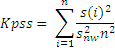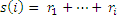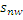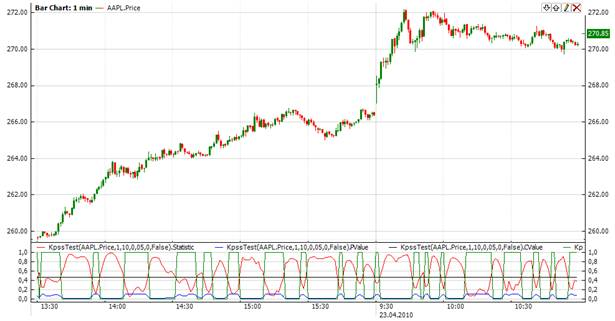﻿KPSSTest

# KPSSTest

The test of Kwiatkowski, Phillips, Schmidt and Shin(KpssTest) assesses the null hypothesis that a univariate time series is trend stationary against the alternative that it is a nonstationary unit-root process. KpssTest is a test for stationarity in a time series. KpssTest indicator returnes 4 values:

CValue – the c-value of the test statistic

Decision – the decision for the test. 1.0 – if the null hypothesis is accepted(series is stationary); 0.0 – if the null hypothesis is rejected(series has unit root)

PValue - the p-value of the test statistic

Statistic – the statistic of KPSS TestCalculation

The test statistic is:whereis the vector of residuals from the regression,is the Newey-West estimator of the long-run variance, and n is the sample size.Chart ExampleImplementation and Usage

To initialize KpssTest test indicator use one of the constructors provided:

KpssTest – this constructor sets default value 1 for updatePeriod, 10 for workingPeriod, 0.05 for significanceLevel, 0 for numberOfLags and false for includeTrend.

KpssTest(Int32, Double, Int32, Boolean) – creates KPSS Test indicator with expanding window

KpssTest(TimeSpan, Double, Int32, Boolean) – creates KPSS Test indicator with expanding window. updatePeriod parameter is of TimeSpan type.

To operate with sliding data sets, it is necessary to define the sliding window size value as a time period or a number of data points in the following constructors(note that updatePeriod can take either TimeSpan or int value):

KpssTest(Int32, Int32, Double, Int32, Boolean)

KpssTest(Int32, TimeSpan, Double, Int32, Boolean)

KpssTest -  initializes an instance of KpssTest with sliding window and update sheduled by point period.  workingPeriod is of TimeSpan type.

KpssTest - initializes an instance of KpssTest with sliding window and update sheduled by time period. workingPeriod is of TimeSpan type.

Use

CValue

PValue

Decision

Statistic

properties to get current value.Example
C#
``` 1// Create new instance
2KpssTest kpss = new KpssTest(5, 20, 0.06, 2, false);
3
4// Number of stored values
5kpss.HistoryCapacity = 2;
6
9
10// Get indicator values
11double cValue = kpss.CValue;
12double pValue = kpss.PValue;
13double decision = kpss.Decision;
14double statistic = kpss.Statistic;
15// Get previous values
16if(kpss.HistoryCount == 2)
17{
18    double PrevCValue = kpss.CValue;
19    double PrevPValue = kpss.PValue;
20    double PrevDecision = kpss.Decision;
21    double PrevStatistic = kpss.Statistic;
22}```